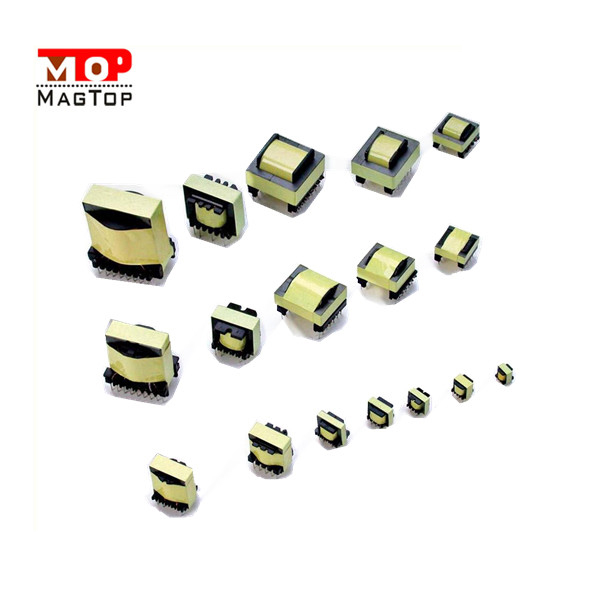# Working principle of high frequency transformer 2

##### author: MagTop
06/06/2022The working principle of high frequency transformer
There are many topologies in switching power supply. For example, in the half-bridge power conversion circuit, two switching triodes turn on in turn to generate high-frequency pulse waves of 100kHz at work, and then change the voltage through the high-frequency transformer to output ac power. The ratio of turns of each winding of the high-frequency transformer determines the output voltage.
A typical half bridge type transformer circuit in the most conspicuous is three high frequency transformer, the main transformer, transformer and auxiliary transformer (standby transformer), each transformer in the regulations of the state has its own measure, such as main transformer, as long as it is more than 200 w power supply, its core diameter (level) shall not be less than 35 mm. And the auxiliary transformer, when the power supply does not exceed 300W, its magnetic core diameter of 16mm is enough.
The main parameters of the transformer are voltage ratio, frequency characteristics, rated power and efficiency.
Voltage ratio N: The relationship between the transformer voltage ratio n and the number of turns and voltage of the primary and secondary windings is as follows: n=V1/V2=N1/N2 In the formula, N1 is the primary (primary) winding of the transformer, N2 is the secondary (secondary) winding, V1 is the voltage at both ends of the primary winding, V2 is the voltage at both ends of the secondary winding.
Step-up transformer voltage ratio n is less than 1, step-down transformer voltage ratio n is greater than 1, isolation transformer voltage ratio is equal to 1.
Rated power P: This parameter is generally used for power transformers. It refers to the power transformer in the specified working frequency and voltage, can work for a long time without exceeding the specified temperature output power.
The rated power of the transformer is related to the cross-sectional area of the core and the diameter of enameled wire. Transformer core cross-sectional area, enameled wire diameter thick, its output power is also large.
Frequency characteristics: refers to the transformer has a certain operating frequency range, different operating frequency range of transformers, generally can not be used interchangeably. Because the transformer has its frequency range of work, there will be working temperature rise or can not work normally.
Efficiency: refers to the ratio of transformer output power to input power at rated load. The value is proportional to the output power of the transformer, that is, the greater the output power of the transformer, the higher the efficiency; The smaller the transformer's output, the less efficient it is.
Transformer efficiency value is generally between 60%~100%.